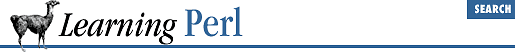home | O'Reilly's CD bookshelfs | FreeBSD | Linux | Cisco | Cisco Exam

### A.7 Chapter 8, Functions

1. Here's one way to do it:

```

sub card {
my %card_map;
@card_map{1..9} = qw(
one two three four five six seven eight nine
);

my(\$num) = @_;
if (\$card_map{\$num}) {
return \$card_map{\$num};
} else {
return \$num;
}
}
# driver routine:
while (

<>) {
chomp;
print "card of \$_ is ", &card(\$_), "\n";
}```

The ``` &card``` subroutine (so named because it returns a cardinal name for a given value) begins by initializing a constant hash called ``` %card_map``` . This array has values such that ``` \$card_map{6}``` is ``` six``` , making it fairly easy to do the mapping.

The ``` if``` statement determines if the value is in range by looking the number up in the hash: if there's a corresponding hash element, the test is true, so that array element is returned. If there's no corresponding element (such as when ``` \$num``` is ``` 11``` or ``` -4``` ), the value returned from the hash lookup is ``` undef``` , so the ``` else``` -branch of the ``` if``` statement is executed, returning the original number. You can also replace that entire ``` if``` statement with the single expression:

`\$card_map{\$num} || \$num;`

If the value on the left of the ``` ||``` is true, it's the value for the entire expression, which then gets returned. If it's false (such as when ``` \$num``` is out of range), the right side of the ``` ||``` operator is evaluated, returning ``` \$num``` as the return value.

The driver routine takes successive lines, chomping off their newlines, and hands them one at a time to the ``` &card``` routine, printing the result.

2. Here's one way to do it:

```sub card { ...; } # from previous problem
print "Enter first number: ";
chomp(\$first = <STDIN>);
print "Enter second number: ";
chomp(\$second = <STDIN>);
\$message = card(\$first)

. " plus " .
card(\$second) . " equals " .
card(\$first+\$second) . ".\n";
print "\u\$message";```

The first two ``` print``` statements prompt for two numbers, with the immediately following statements reading the values into ``` \$first``` and ``` \$second``` .

A string called ``` \$message``` is then built up by calling ``` &card``` three times, once for each value and once for the sum.

Once the message is constructed, its first character is uppercased by the case-shifting backslash operator ``` \u``` . The message is then printed.

3. Here's one way to do it:

```sub card {
my %card_map;
@card_map{0..9} = qw(
zero one two three four five six seven eight nine
);

my(\$num) = @_;
my(\$negative);
if (\$num < 0) {
\$negative = "negative ";
\$num = - \$num;
}
if (\$card_map{\$num}) {
return \$negative . \$card_map{\$num};
} else {
return \$negative . \$num;
}
}```

Here, we've given the ``` %card_map``` array a name for zero.

The first ``` if``` statement inverts the sign of ``` \$num``` and sets ``` \$negative``` to the word negative, if the number is found to be less than zero. After this ``` if``` statement, the value of ``` \$num``` is always nonnegative, but we will have an appropriate prefix string in ``` \$negative``` .

The second ``` if``` statement determines if the (now positive) ``` \$num``` is within the hash. If so, the resulting hash value is appended to the prefix within ``` \$negative``` and returned. If not, the value within ``` \$negative``` is attached to the original number.

That last ``` if``` statement can be replaced with the expression:

`  \$negative . (\$card_map{\$num} || \$num);`A.6 Chapter 7, Regular ExpressionsA.8 Chapter 9, Miscellaneous Control Structures